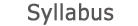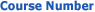0366-1130 Linear Algebra 1c2019 - 2020View groups
Course description

Linear systems of equations, Gauss elimination, homogeneous systems. Matrices- classification, operations, inverse matrices, determinants. Vector spaces: operations on vectors, linear dependence, bases and dimension, change of base, subspaces, scalar and vector multiplication, orthogonal bases, projection. Linear transformations, kernel and image, matrix of a transformation, one-to-one and inverse transformations, change of base. Eigenvalues and eigenvectors, algebraic and geometric multiplicity, diagonalization of a matrix.

accessibility declaration